# NCERT Solutions for Class 9 Maths chapter 13 Exercise 13.2 - Surface Areas and Volumes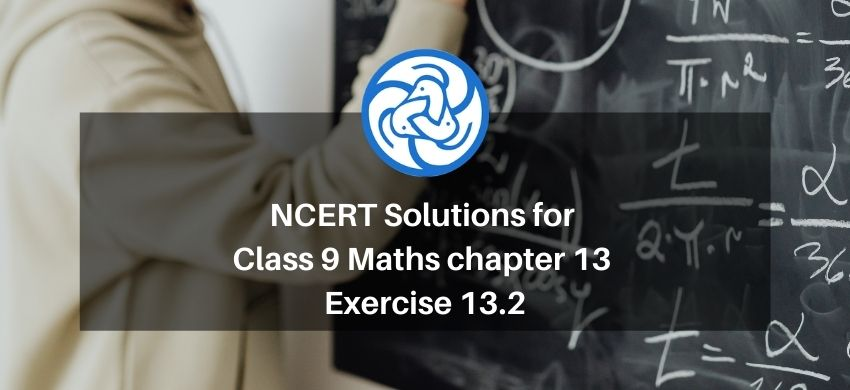Hey, are you a class 9 Student and Looking for Ways to Download NCERT Solutions for Class 9 Maths chapter 13 Exercise 13.2? If Yes then you are at the right place.

Here we have listed Class 9 maths chapter 13 exercise 13.2 solutions in PDF that is prepared by Kota’s top IITian’s Faculties by keeping Simplicity in mind.

If you want to score high in your class 9 Maths Exam then it is very important for you to have a good knowledge of all the important topics, so to learn and practice those topics you can use eSaral NCERT Solutions.

So, without wasting more time Let’s start.

### Download The PDF of NCERT Solutions for Class 9 Maths chapter 13 Exercise 13.2 "Surface Areas and Volumes"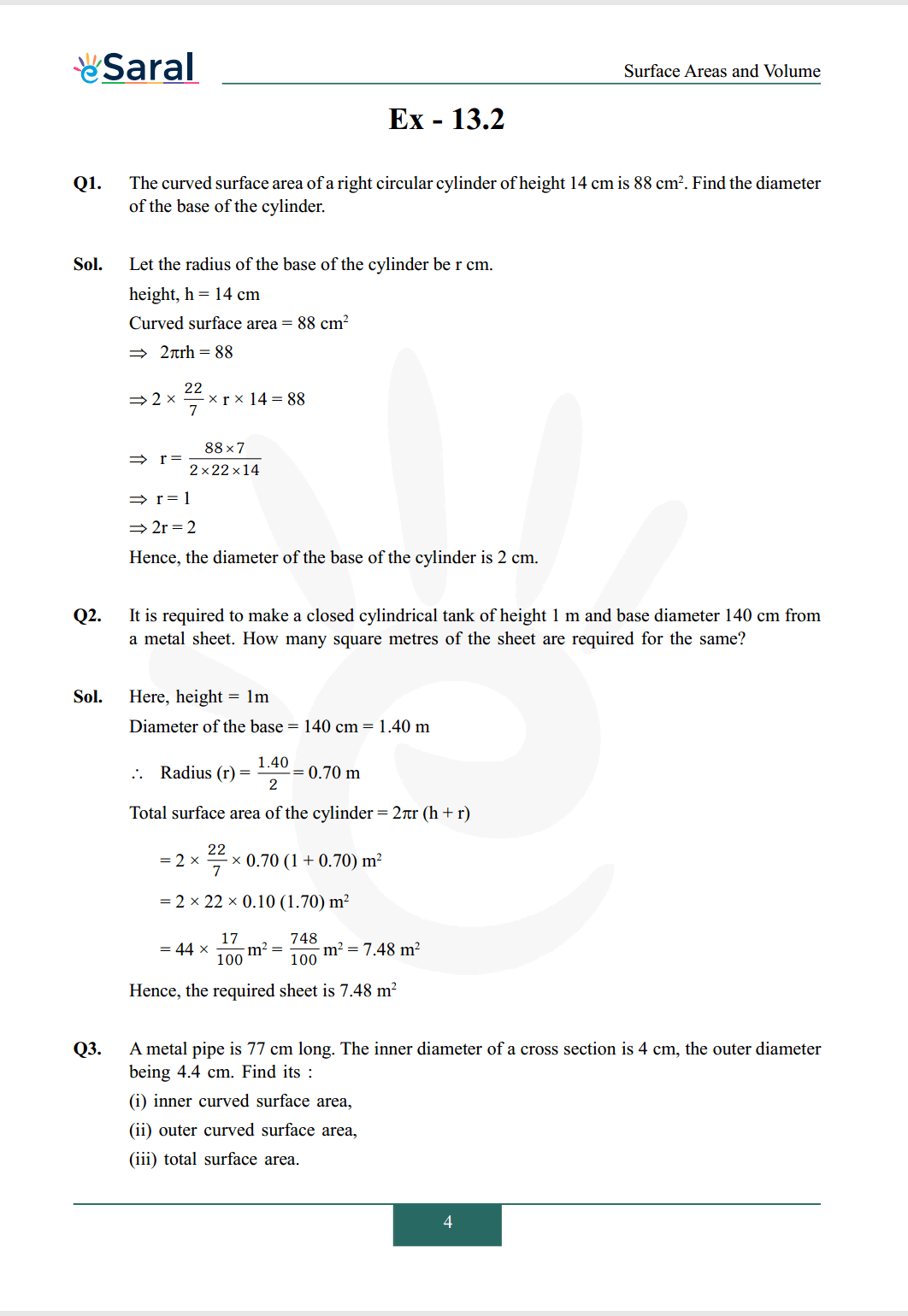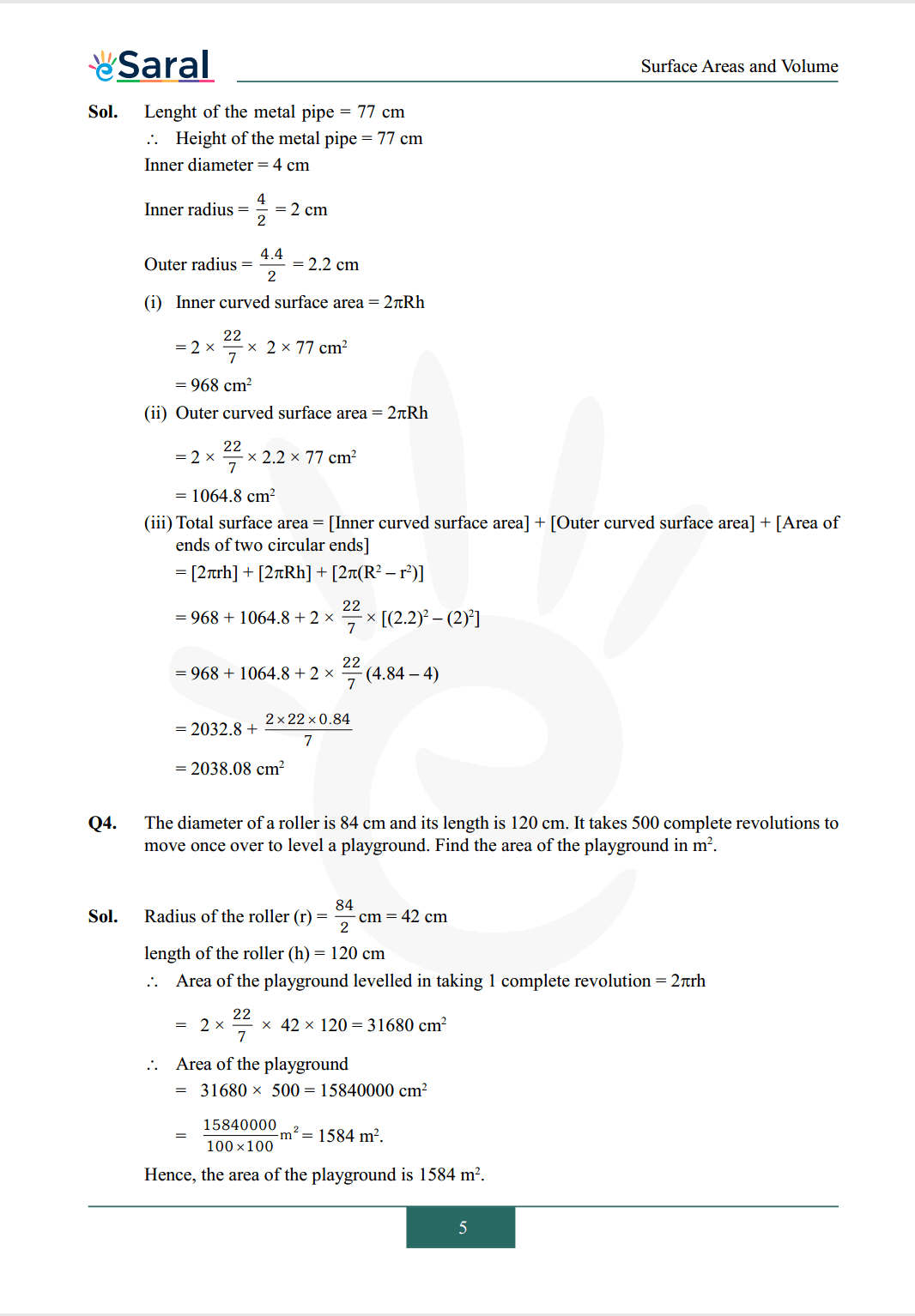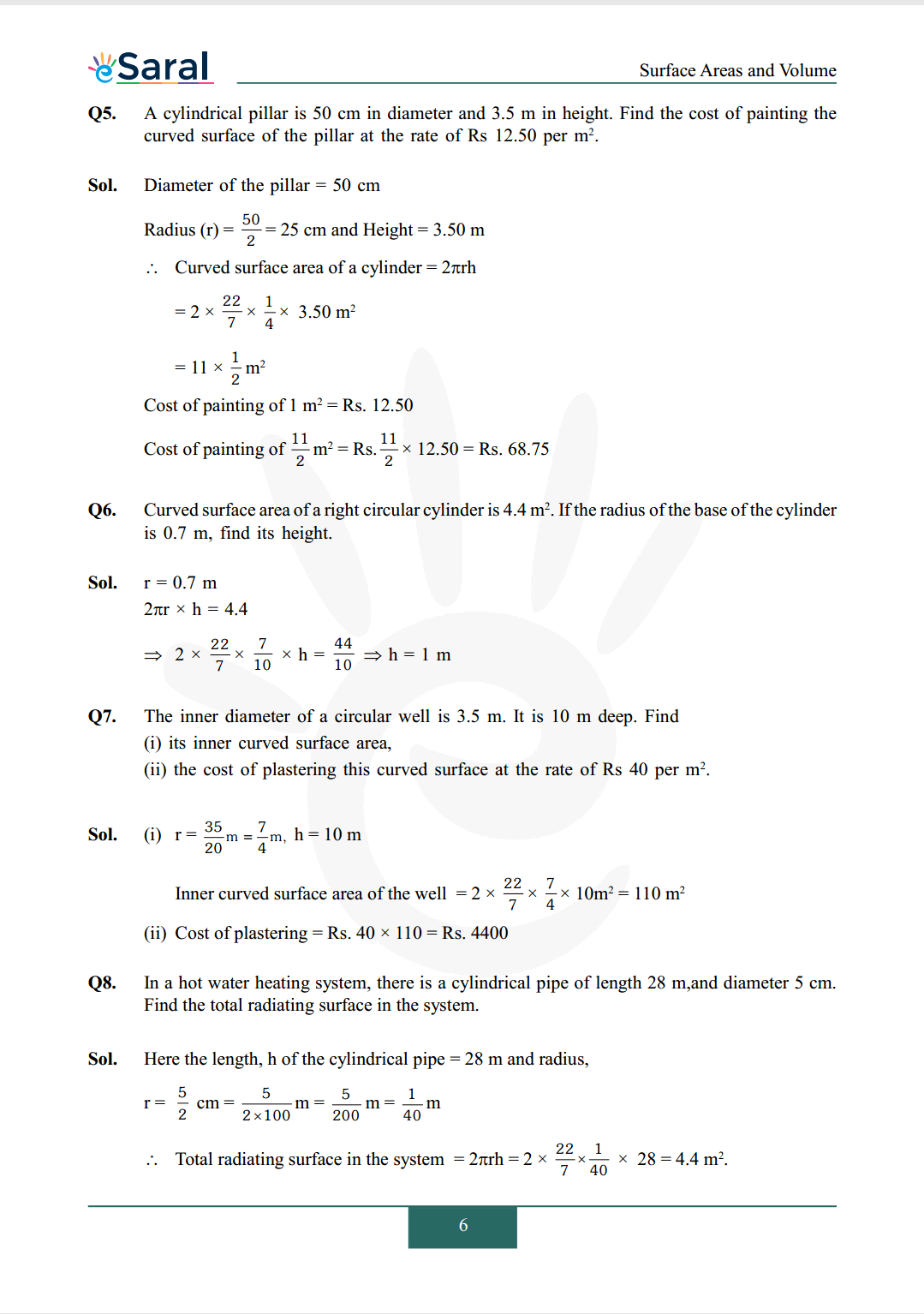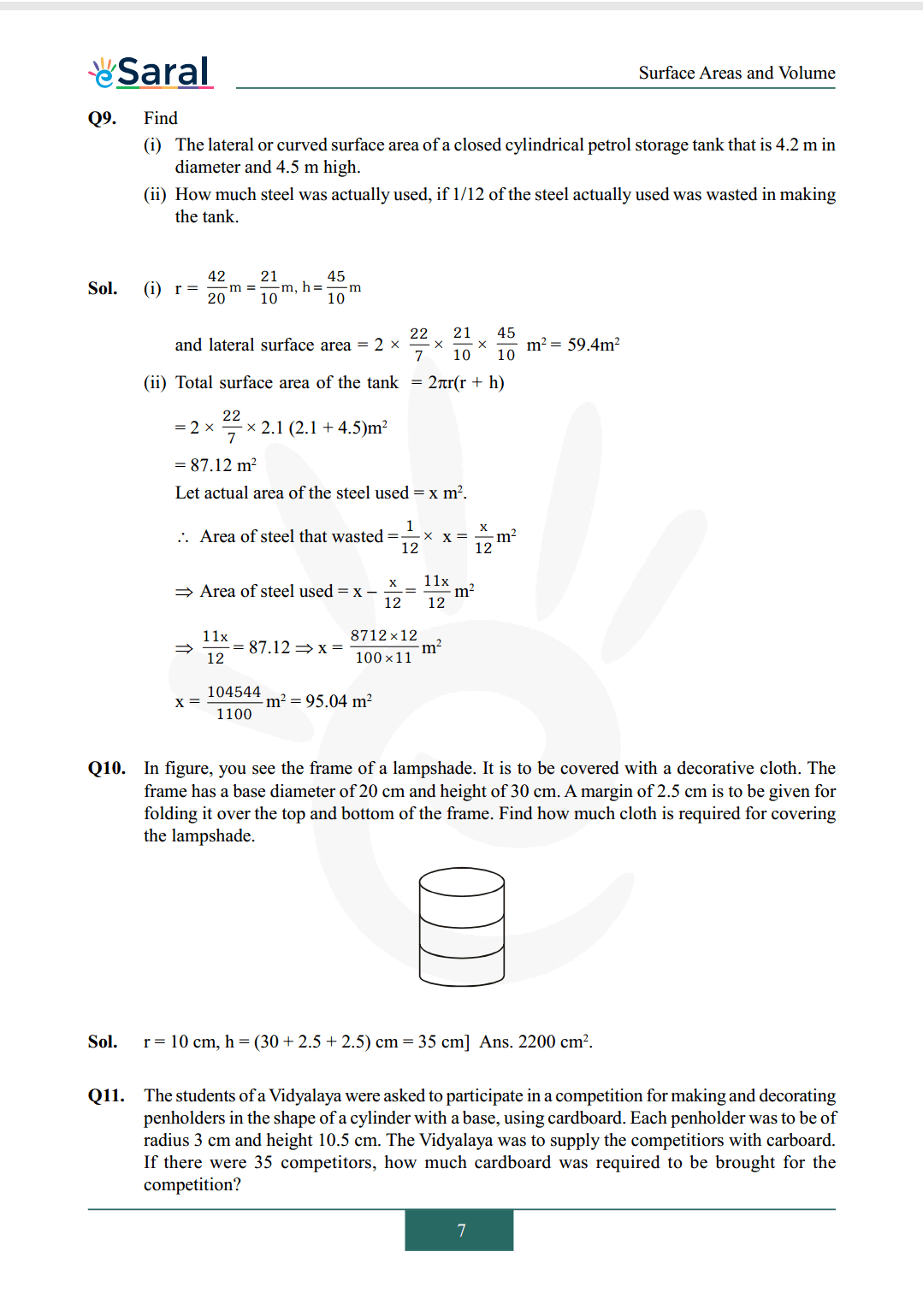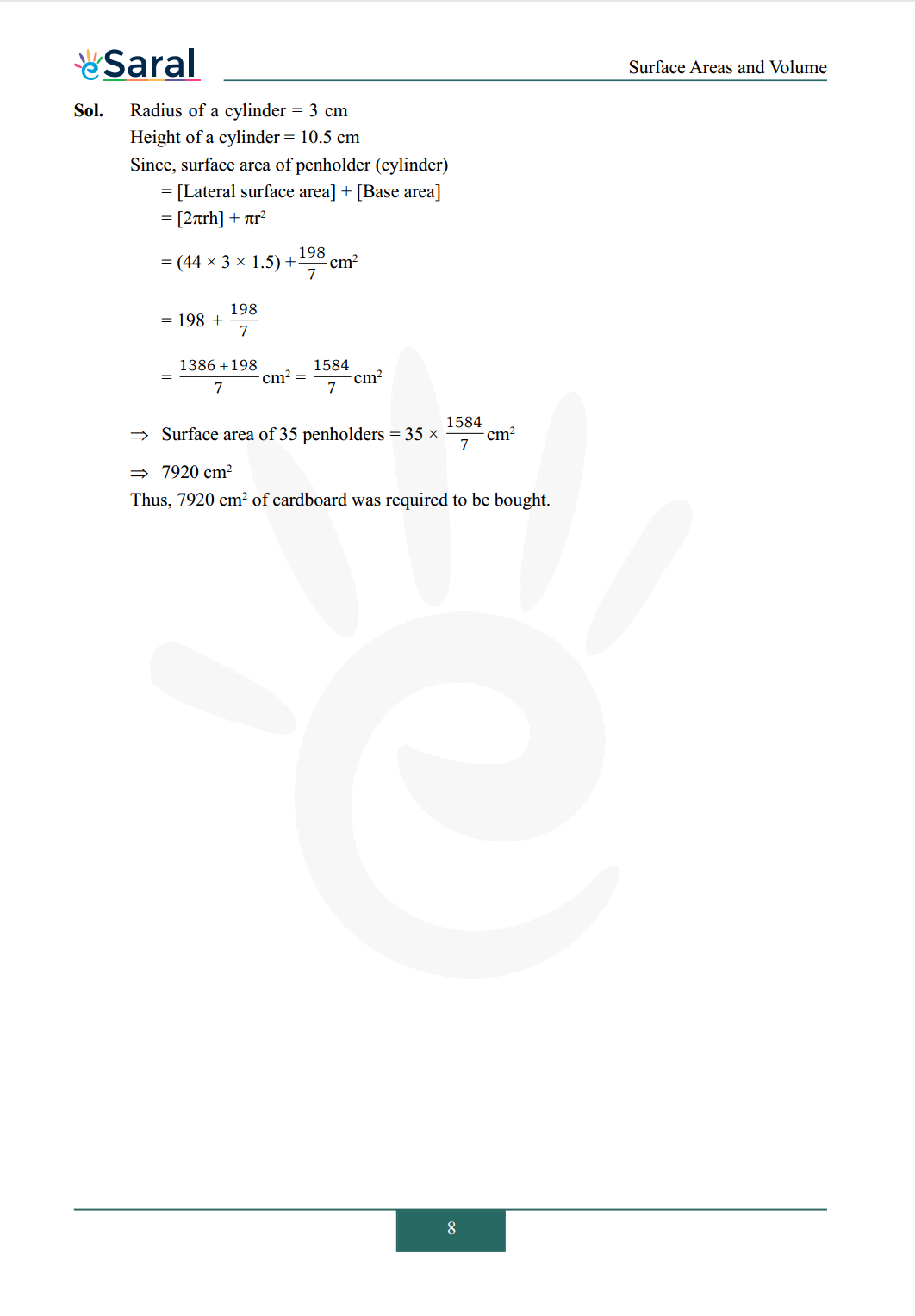#### All Questions of Chapter 13 Exercise 13.2

Once you complete the chapter 13 then you can revise Ex. 13.2 by solving following questions

Q1. The curved surface area of a right circular cylinder of height $14 \mathrm{~cm}$ is $88 \mathrm{~cm}^{2}$. Find the diameter of the base of the cylinder.

Q2. It is required to make a closed cylindrical tank of height 1 m and base diameter 140 cm from a metal sheet. How many square metres of the sheet are required for the same?

Q3. A metal pipe is $77 \mathrm{~cm}$ long. The inner diameter of a cross section is $4 \mathrm{~cm}$, the outer diameter being $4.4 \mathrm{~cm}$. Find its :
(i) inner curved surface area,
(ii) outer curved surface area,
(iii) total surface area.

Q4. The diameter of a roller is $84 \mathrm{~cm}$ and its length is $120 \mathrm{~cm}$. It takes 500 complete revolutions to move once over to level a playground. Find the area of the playground in $\mathrm{m}^{2}$.

Q5. A cylindrical pillar is $50 \mathrm{~cm}$ in diameter and $3.5 \mathrm{~m}$ in height. Find the cost of painting the curved surface of the pillar at the rate of Rs $12.50$ per $\mathrm{m}^{2}$.

Q6. Curved surface area of a right circular cylinder is $4.4 \mathrm{~m}^{2}$. If the radius of the base of the cylinder is $0.7 \mathrm{~m}$, find its height.

Q7. The inner diameter of a circular well is $3.5 \mathrm{~m}$. It is $10 \mathrm{~m}$ deep. Find
(i) its inner curved surface area,

Q8. In a hot water heating system, there is a cylindrical pipe of length $28 \mathrm{~m}$, and diameter $5 \mathrm{~cm}$. Find the total radiating surface in the system.

Q9. Find
(i) The lateral or curved surface area of a closed cylindrical petrol storage tank that is $4.2 \mathrm{~m}$ in diameter and $4.5 \mathrm{~m}$ high.
(ii) How much steel was actually used, if $1 / 12$ of the steel actually used was wasted in making the tank.

Q10. In figure, you see the frame of a lampshade. It is to be covered with a decorative cloth. The frame has a base diameter of $20 \mathrm{~cm}$ and height of $30 \mathrm{~cm}$. A margin of $2.5 \mathrm{~cm}$ is to be given for folding it over the top and bottom of the frame. Find how much cloth is required for covering the lampshade.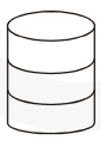Q11. The students of a Vidyalaya were asked to participate in a competition for making and decorating penholders in the shape of a cylinder with a base, using cardboard. Each penholder was to be of radius $3 \mathrm{~cm}$ and height $10.5 \mathrm{~cm}$. The Vidyalaya was to supply the competitiors with carboard. If there were 35 competitors, how much cardboard was required to be brought for the competition?

NCERT Class 9 Maths Book

NCERT Class 9 Maths Exemplar

Complete Solutions for Class 9 Maths chapter 13

Class 9 Maths Chapter 12 Exercise 12.1 Solutions

Class 9 Maths Chapter 12 Exercise 12.2 Solutions

If you have any Confusion related to NCERT Solutions for Class 9 Maths chapter 13 Exercise 13.2 then feel free to ask in the comments section down below.

To watch Free Learning Videos on Class 9 by Kota’s top Faculties Install the eSaral App